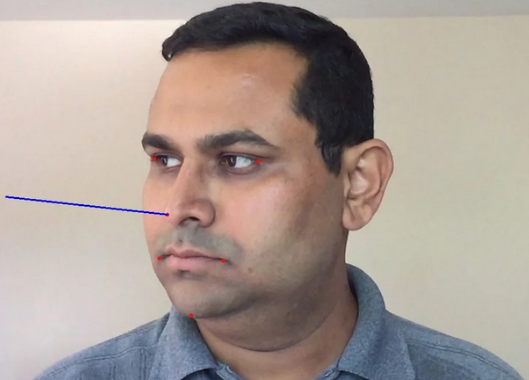## 2. 相机运动(camera motion) 的数学描述

3D 刚体仅有两种相对于相机的运动：

 - 平移

 - 旋转

## 3. 姿态估计所需信息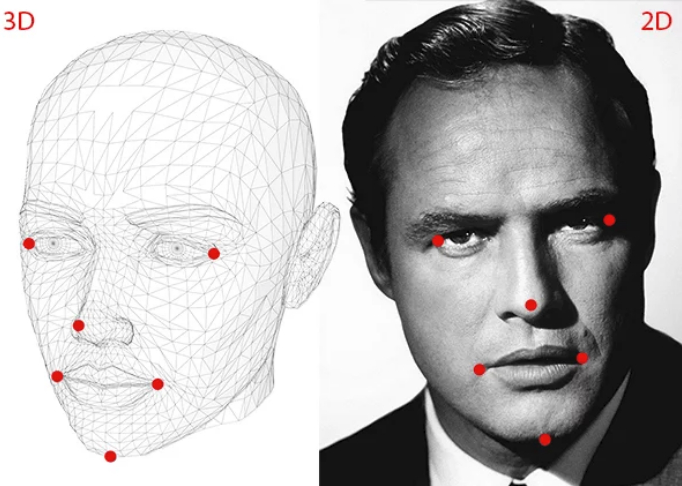- 一些点的 2D 坐标位置

 - 同一批点的 3D 位置

• (1) - 鼻尖：( 0.0, 0.0, 0.0)
• (2) - 下巴：( 0.0, -330.0, -65.0)
• (3) - 左眼左角：(-225.0f, 170.0f, -135.0)
• (4) - 右眼右角：( 225.0, 170.0, -135.0)
• (5) - 左嘴角：(-150.0, -150.0, -125.0)
• (6) - 右嘴角：(150.0, -150.0, -125.0)

 - 相机的内在参数(intrinsic parameters)

## 4. 姿态估计算法原理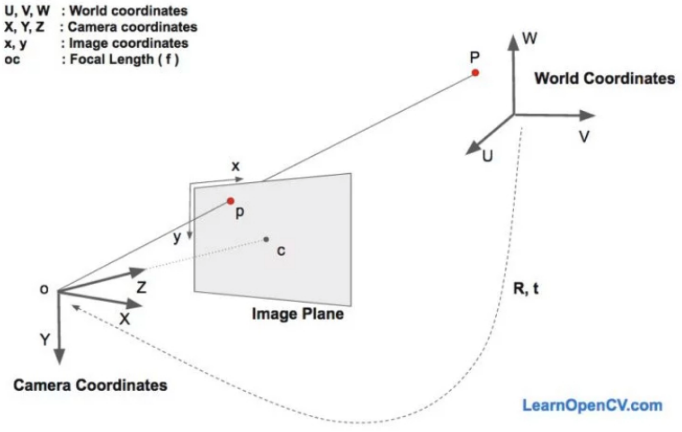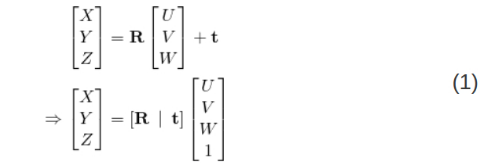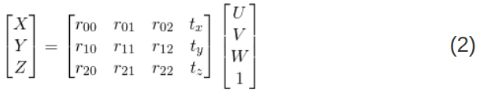## 5. 直接线性变换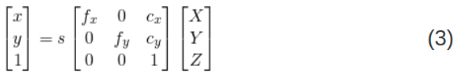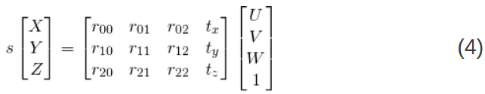## 6. Levenberg-Marquardt Optimization

DLT 方法可能是不够精确的，其主要原因是：

 - 旋转 $\mathbf{R}$ 包含三个自由度，但 DLT 算法中的矩阵表示要采用 9 个数字. 并没有限制该估计的 3x3 矩阵必须为旋转矩阵.

 - 更重要的是，DLT 方法并不是对正确的目标函数进行最小化. 理想情况下，想要最小化的是重投影误差(reprojection error).

## 7. OpenCV solvePnP 函数

OpenCV 中，solvePnP 函数和 solvePnPRansac 函数可以用于估计姿态.

solvePnP 函数实现了几种姿态估计算法，可以根据参数 flag 进行选择. 默认 flagSOLVEPNP_ITERATIVE，其是采用 Levenberg-Marquardt 优化的 DTL 方案；SOLVEPNP_P3P 仅采用 3 个点用于计算姿态，只有在采用 solvePnPRansac 函数时才被使用.

OpenCV 3中，包含了新的算法，SOLVEPNP_DLSSOLVEPNP_UPNP. 其中，有意思的是，SOLVEPNP_UPNP 还尝试估计相机的内在参数.

// C++:
bool solvePnP(InputArray objectPoints,
InputArray imagePoints,
InputArray cameraMatrix,
InputArray distCoeffs,
OutputArray rvec,
OutputArray tvec,
bool useExtrinsicGuess=false,
int flags=SOLVEPNP_ITERATIVE )
# Python:
cv2.solvePnP(
objectPoints,
imagePoints,
cameraMatrix,
distCoeffs[,
rvec[,
tvec[,
useExtrinsicGuess[, flags]
]
]
]
) → retval, rvec, tvec

objectPoints - 世界坐标系空间的物体点数组. 一般是 N 个 3D 点的向量(推荐). 也可以是 Nx3 (或 3xN) 的单通道矩阵，或者 Nx1(或 1xN) 的 3 通道矩阵.

imagePoints - 对应的图像点数组. 一般可以是 N 个 2D 点的向量. 也可以是 Nx2 (或 2xN) 的单通道矩阵，或者 Nx1(或 1xN) 的 2 通道矩阵.

cameraMatrix - 输入的相机矩阵:

$$\begin{bmatrix} f_x & 0 & c_x \\ 0 & f_x & c_y \\ 0 & 0 & 1 \end{bmatrix}$$

distCoeffs - 包含 4， 5， 8 或12 个元素的畸变相关系数的输入向量 $(k_1, k_2, p_1, p_2[, k_3[, k_4, k_5, k_6], [s_1, s_2, s_3, s_4]])$ . 如果该向量为 NULL 或空，则假设是 0 畸变的. 通常该值为 NULL.

rvec - 输出的旋转向量

tvec - 输出的平移向量

useExtrinsicGuess - SOLVEPNP_ITERATIVE 涉及的参数. 如果该值为 True，则函数会采用提供的 rvec 和 tvec 值分别作为旋转向量和平移向量的初始化逼近，再进行进一步的优化.

flags - PnP 问题求解方法：

SOLVEPNP_ITERATIVE Iterative method is based on Levenberg-Marquardt optimization. In this case, the function finds such a pose that minimizes reprojection error, that is the sum of squared distances between the observed projections imagePoints and the projected (using projectPoints() ) objectPoints .

SOLVEPNP_P3P Method is based on the paper of X.S. Gao, X.-R. Hou, J. Tang, H.-F. Chang “Complete Solution Classification for the Perspective-Three-Point Problem”. In this case, the function requires exactly four object and image points.

SOLVEPNP_EPNP Method has been introduced by F.Moreno-Noguer, V.Lepetit and P.Fua in the paper “EPnP: Efficient Perspective-n-Point Camera Pose Estimation”.

The flags below are only available for OpenCV 3

SOLVEPNP_DLS Method is based on the paper of Joel A. Hesch and Stergios I. Roumeliotis. “A Direct Least-Squares (DLS) Method for PnP”.

SOLVEPNP_UPNP Method is based on the paper of A.Penate-Sanchez, J.Andrade-Cetto, F.Moreno-Noguer. “Exhaustive Linearization for Robust Camera Pose and Focal Length Estimation”. In this case the function also estimates the parameters f_x and f_y assuming that both have the same value. Then the cameraMatrix is updated with the estimated focal length.

## 8. OpenCV solvePnPRansac 函数

solvePnPRansac 函数与 solvePnP 函数非常相似，除了其采用了 Random Sample Consensus ( RANSAC ) 以进行更鲁棒的姿态估计.

// C++:
void solvePnPRansac(InputArray objectPoints,
InputArray imagePoints,
InputArray cameraMatrix,
InputArray distCoeffs,
OutputArray rvec,
OutputArray tvec,
bool useExtrinsicGuess=false,
int iterationsCount=100,
float reprojectionError=8.0,
int minInliersCount=100,
OutputArray inliers=noArray(),
int flags=ITERATIVE)
# Python:
cv2.solvePnPRansac(
objectPoints,
imagePoints,
cameraMatrix,
distCoeffs[,
rvec[,
tvec[,
useExtrinsicGuess[,
iterationsCount[,
reprojectionError[,
minInliersCount[,
inliers[, flags]
]
]
]
]
]
]
]
) → rvec, tvec, inliers

iterationsCount – The number of times the minimum number of points are picked and the parameters estimated.
reprojectionError – As mentioned earlier in RANSAC the points for which the predictions are close enough are called “inliers”. This parameter value is the maximum allowed distance between the observed and computed point projections to consider it an inlier.
minInliersCount – Number of inliers. If the algorithm at some stage finds more inliers than minInliersCount , it finishes.
inliers – Output vector that contains indices of inliers in objectPoints and imagePoints .

## 9. OpenCV POSIT

OpenCV 早期采用了 POSIT 的姿态估计算法，其是 C 代码的 API (cvPosit)，并不是 C++ API 的一部分.

## 10. OpenCV 姿态估计的实现源码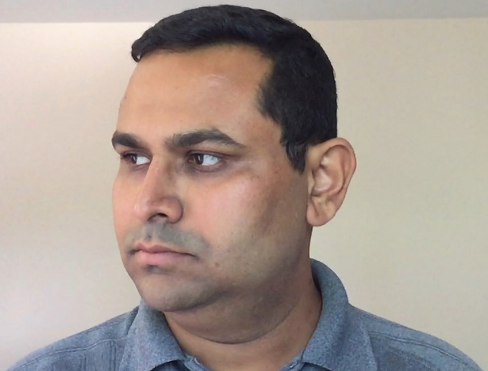### 10.1. C++ 实现

#include <opencv2/opencv.hpp>

using namespace std;
using namespace cv;

int main(int argc, char **argv)
{

// 2D image points. If you change the image, you need to change vector
std::vector<cv::Point2d> image_points;
image_points.push_back( cv::Point2d(359, 391) );    // Nose tip
image_points.push_back( cv::Point2d(399, 561) );    // Chin
image_points.push_back( cv::Point2d(337, 297) );     // Left eye left corner
image_points.push_back( cv::Point2d(513, 301) );    // Right eye right corner
image_points.push_back( cv::Point2d(345, 465) );    // Left Mouth corner
image_points.push_back( cv::Point2d(453, 469) );    // Right mouth corner

// 3D model points.
std::vector<cv::Point3d> model_points;
model_points.push_back(cv::Point3d(0.0f, 0.0f, 0.0f));               // Nose tip
model_points.push_back(cv::Point3d(0.0f, -330.0f, -65.0f));          // Chin
model_points.push_back(cv::Point3d(-225.0f, 170.0f, -135.0f));       // Left eye left corner
model_points.push_back(cv::Point3d(225.0f, 170.0f, -135.0f));        // Right eye right corner
model_points.push_back(cv::Point3d(-150.0f, -150.0f, -125.0f));      // Left Mouth corner
model_points.push_back(cv::Point3d(150.0f, -150.0f, -125.0f));       // Right mouth corner

// Camera internals
double focal_length = im.cols; // Approximate focal length.
Point2d center = cv::Point2d(im.cols/2,im.rows/2);
cv::Mat camera_matrix = (cv::Mat_<double>(3,3) << focal_length, 0, center.x, 0 , focal_length, center.y, 0, 0, 1);
cv::Mat dist_coeffs = cv::Mat::zeros(4,1,cv::DataType<double>::type); // Assuming no lens distortion

cout << "Camera Matrix " << endl << camera_matrix << endl ;
// Output rotation and translation
cv::Mat rotation_vector; // Rotation in axis-angle form
cv::Mat translation_vector;

// Solve for pose
cv::solvePnP(model_points, image_points, camera_matrix, dist_coeffs, rotation_vector, translation_vector);

// Project a 3D point (0, 0, 1000.0) onto the image plane.
// We use this to draw a line sticking out of the nose

vector<Point3d> nose_end_point3D;
vector<Point2d> nose_end_point2D;
nose_end_point3D.push_back(Point3d(0,0,1000.0));

projectPoints(nose_end_point3D, rotation_vector, translation_vector, camera_matrix, dist_coeffs, nose_end_point2D);

for(int i=0; i < image_points.size(); i++)
{
circle(im, image_points[i], 3, Scalar(0,0,255), -1);
}

cv::line(im,image_points, nose_end_point2D, cv::Scalar(255,0,0), 2);

cout << "Rotation Vector " << endl << rotation_vector << endl;
cout << "Translation Vector" << endl << translation_vector << endl;

cout <<  nose_end_point2D << endl;

// Display image.
cv::imshow("Output", im);
cv::waitKey(0);

}

### 10.2. Python 实现

#!/usr/bin/env python
import cv2
import numpy as np
import matplotlib.pyplot as plt

size = im.shape

# 2D image points. If you change the image, you need to change vector
image_points = np.array([
(359, 391),  # Nose tip
(399, 561),  # Chin
(337, 297),  # Left eye left corner
(513, 301),  # Right eye right corne
(345, 465),  # Left Mouth corner
(453, 469)  # Right mouth corner
], dtype="double")

# 3D model points.
model_points = np.array([
(0.0, 0.0, 0.0),  # Nose tip
(0.0, -330.0, -65.0),  # Chin
(-225.0, 170.0, -135.0),  # Left eye left corner
(225.0, 170.0, -135.0),  # Right eye right corne
(-150.0, -150.0, -125.0),  # Left Mouth corner
(150.0, -150.0, -125.0)  # Right mouth corner
])

# Camera internals
focal_length = size
center = (size / 2, size / 2)
camera_matrix = np.array(
[[focal_length, 0, center],
[0, focal_length, center],
[0, 0, 1]], dtype="double")

print("Camera Matrix :\n {0}".format(camera_matrix))

dist_coeffs = np.zeros((4, 1))  # Assuming no lens distortion
(success, rotation_vector, translation_vector) = \
cv2.solvePnP(model_points,
image_points,
camera_matrix,
dist_coeffs,
flags=cv2.cv2.SOLVEPNP_ITERATIVE) #

print("Rotation Vector:\n {0}".format(rotation_vector))
print("Translation Vector:\n {0}".format(translation_vector))

# Project a 3D point (0, 0, 1000.0) onto the image plane.
# We use this to draw a line sticking out of the nose

(nose_end_point2D, jacobian) = cv2.projectPoints(
np.array([(0.0, 0.0, 1000.0)]),
rotation_vector,
translation_vector,
camera_matrix,
dist_coeffs)

for p in image_points:
cv2.circle(im, (int(p), int(p)), 3, (0, 0, 255), -1)

p1 = (int(image_points), int(image_points))
p2 = (int(nose_end_point2D), int(nose_end_point2D))

cv2.line(im, p1, p2, (255, 0, 0), 2)

# Display image
plt.imshow(im[:, :,::-1])
plt.axis("off")
plt.show()

Camera Matrix :
[[1.20e+03 0.00e+00 6.00e+02]
[0.00e+00 1.20e+03 3.37e+02]
[0.00e+00 0.00e+00 1.00e+00]]
Rotation Vector:
[[-0.0581844 ]
[ 2.20231074]
[ 0.01887552]]
Translation Vector:
[[  449.53365497]
[  -95.72475146]
[-2344.07917989]]Last modification：April 24th, 2019 at 09:06 pm